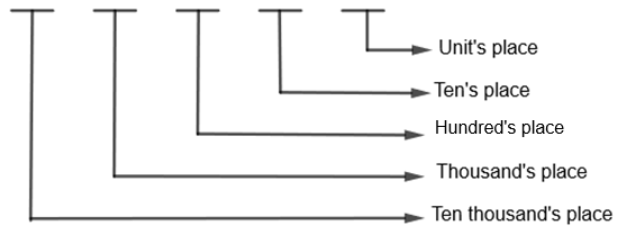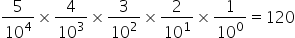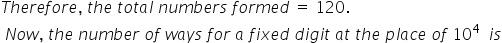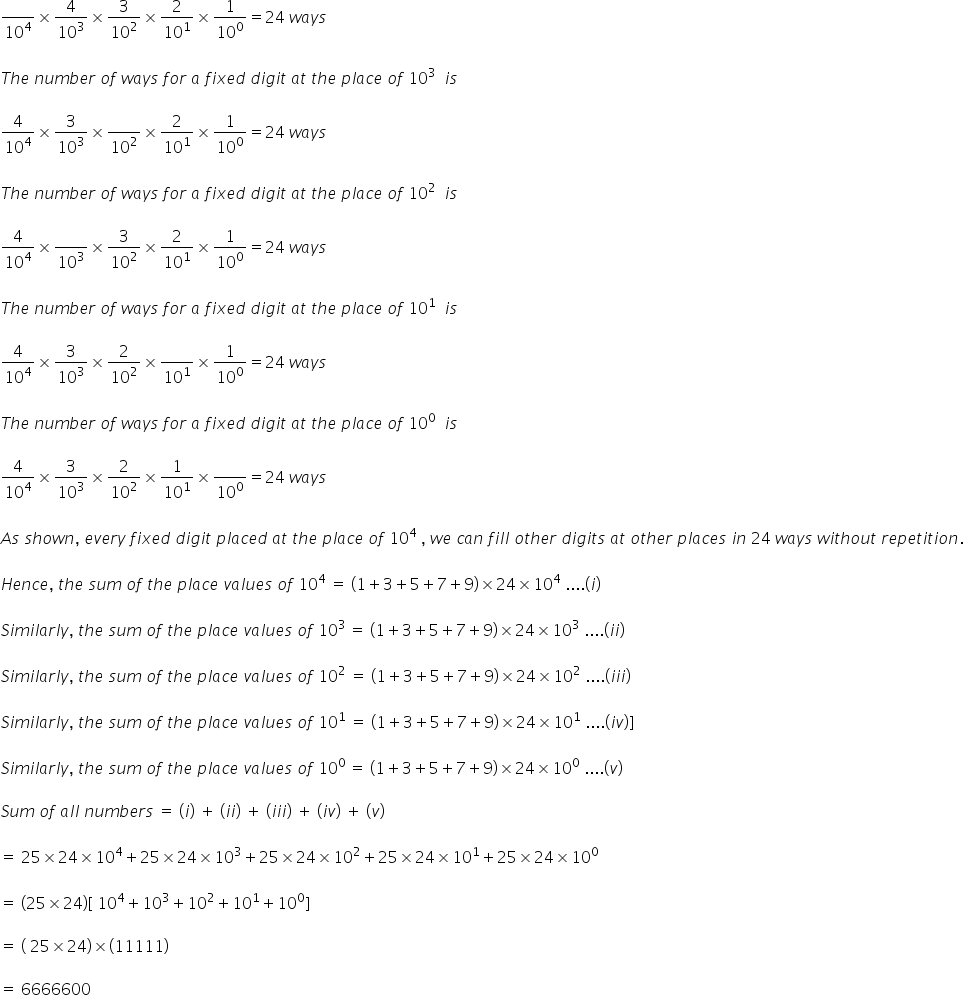Maths-
General
Easy

Question

# The sum of all possible numbers greater than 10,000 formed by using {1,3,5,7,9} is

## 6666600    932460    6660000    1968Hint:

## The correct answer is: 6666600

### Detailed SolutionIn this, we have to find the sum of all numbers greater than 10000 formed by using digits 1, 3, 5, 7, 9, no digit being repeated in any number. We know that any number greater than 10000 would be of 5 digits or more than 5 digits. Also, since we have only 1, 3, 5, 7, 9, and repetition is not allowed, we can make only 5 digit numbers and any number formed by these five digits would be greater than 10000.Any of the five digits can occupy ten thousand places. Since repetition is not allowed any of the remaining 4 digits can occupy a thousand’s place. Similarly, any of the remaining 3 digits can occupy a hundred’s place. Now, ten’s and unit’s place can be occupied by any of the remaining two digits and last remaining digit respectively.Therefore, we get the sum of total numbers greater than 10000 formed by using digits 1, 3, 5, 7, 9 without repetition is 6666600.

Here, some students randomly select the numbers and try to add them which is very lengthy as there are 120 numbers and can lead to mistakes. Also, some students do not consider the place value but in questions such as addition, subtraction, etc. of numbers, place value method is most promising.#### With Turito Foundation.#### Get an Expert Advice From Turito.